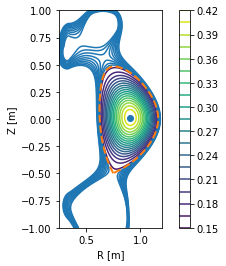# Basic example¶

The following example shows how to load an equilibrium saved in the EQDSK format and perform some basic operations with it. Several test equilibria come shipped with PLEQUE; here we will use onee of them.

:

from pleque.io import readers
import pkg_resources
import matplotlib as plt

#Locate the test equilibrium
filepath = pkg_resources.resource_filename('pleque', 'resources/baseline_eqdsk')


The heart of PLEQUE is its Equilibrium class, which contains all the equilibrium information (and much more). Typically its instances are called eq.

:

# Create an instance of the Equilibrium class

  nx = 65, ny = 129
197 1
---------------------------------
Equilibrium module initialization
---------------------------------
--- Generate 2D spline ---
--- Looking for critical points ---
--- Recognizing equilibrium type ---
>> X-point plasma found.
--- Looking for LCFS: ---
Relative LCFS error: 4.626463974600894e-12
--- Generate 1D splines ---
--- Mapping midplane to psi_n ---
--- Mapping pressure and f func to psi_n ---


The Equilibrium class comes with many interesting functions and caveats.

:

# Plot a simple overview of the equilibrium
eq.plot_overview()

# Calculate the separatrix area
sep_area = eq.lcfs.area
print('Separatrix area: A_sep = %.3f m^2' % sep_area)

# Get absolute magnetic field magnitude at given point
R = 0.7 #m
Z = 0.1 #m
B = eq.B_abs(R, Z)
print('Magnetic field at R=%.1f m and Z=%.1f m: B = %.1f T' % (R, Z, B))

Matplotlib is building the font cache; this may take a moment.

Separatrix area: A_sep = 0.381 m^2
Magnetic field at R=0.7 m and Z=0.1 m: B = 6.7 TBrowse various attributes and functions of the Equilibrium class to see what it has to offer.